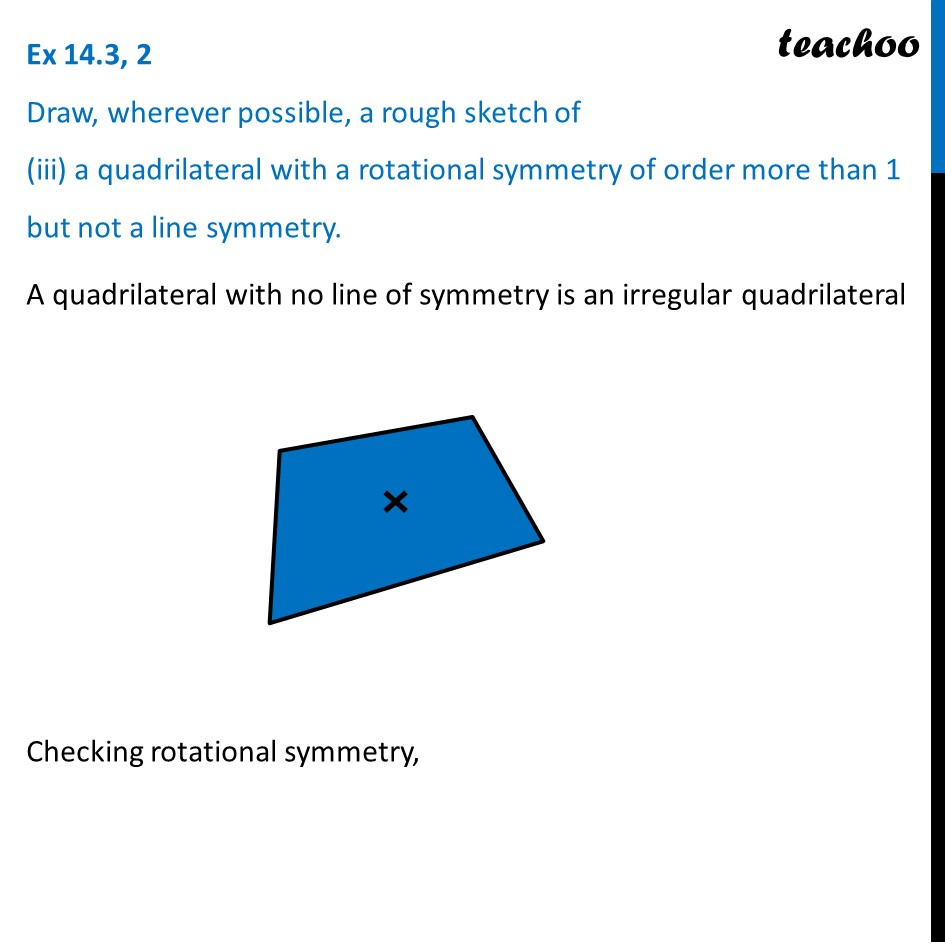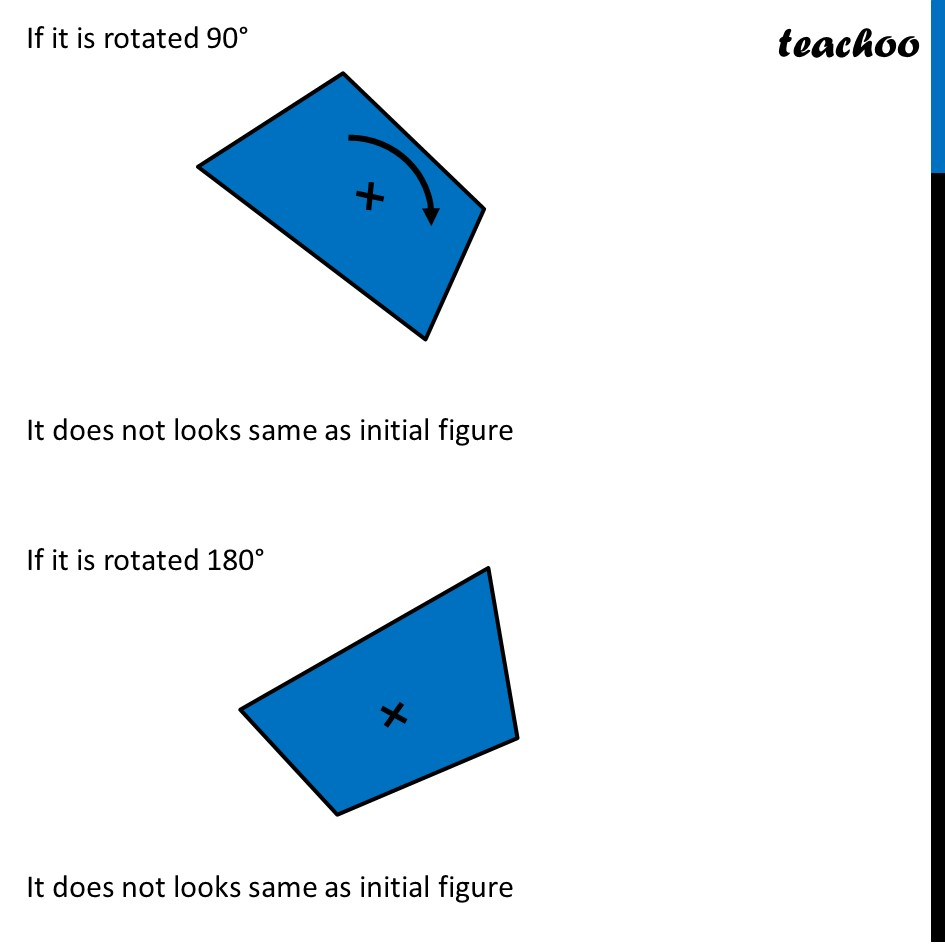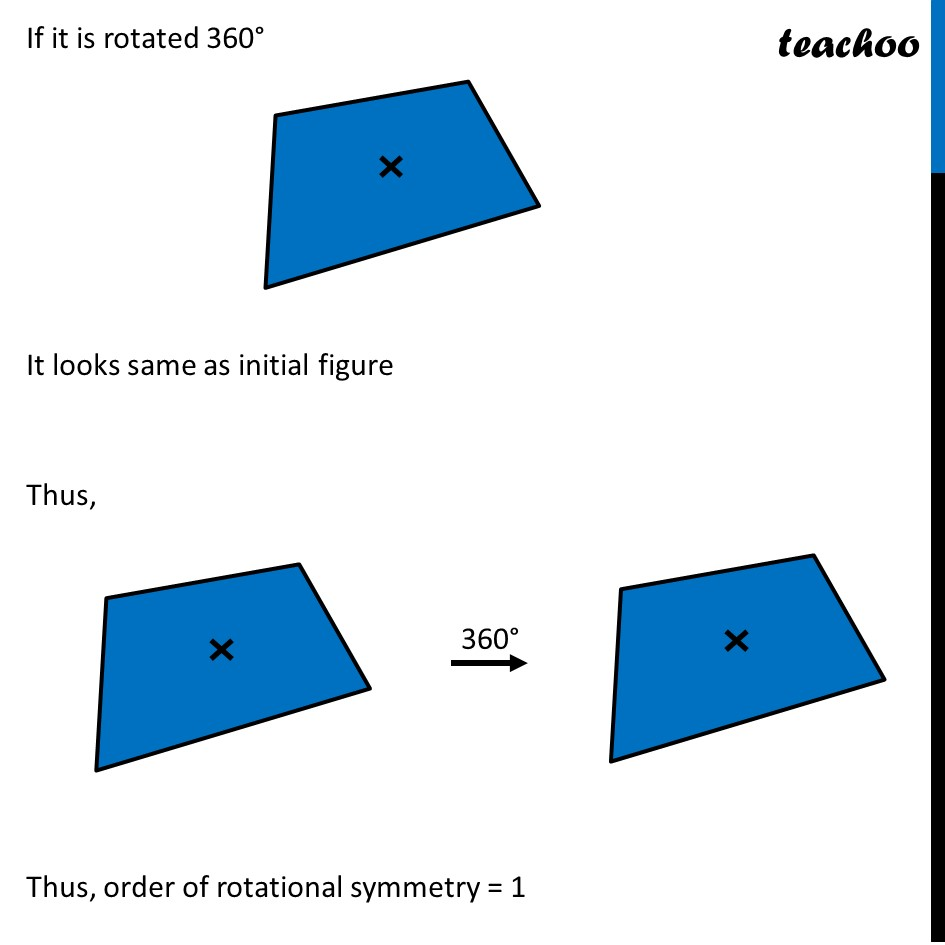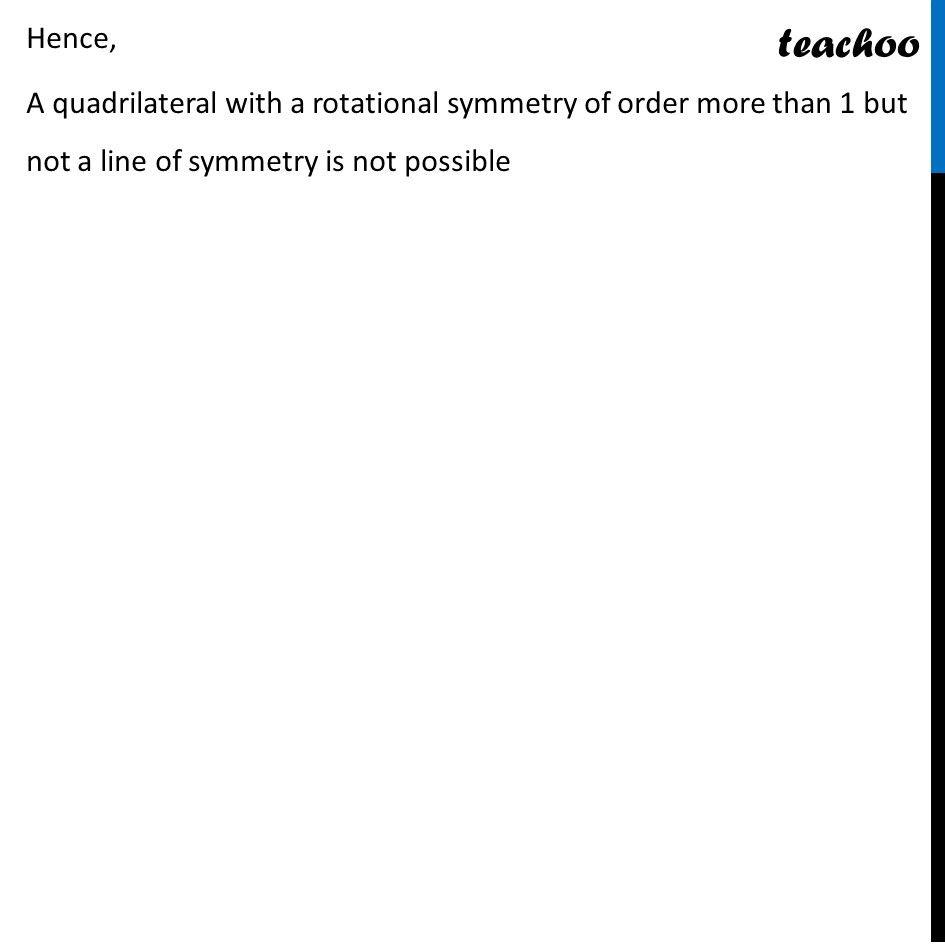Lines and Rotational symmetry (Both)

Chapter 14 Class 7 Symmetry
Concept wiseIntroducing your new favourite teacher - Teachoo Black, at only ₹83 per month

### Transcript

Ex 14.3, 2 Draw, wherever possible, a rough sketch of (iii) a quadrilateral with a rotational symmetry of order more than 1 but not a line symmetry.A quadrilateral with no line of symmetry is an irregular quadrilateral Checking rotational symmetry, If it is rotated 90° It does not looks same as initial figure If it is rotated 180° It does not looks same as initial figure If it is rotated 360° It looks same as initial figure Thus, Thus, order of rotational symmetry = 1 Hence, A quadrilateral with a rotational symmetry of order more than 1 but not a line of symmetry is not possible